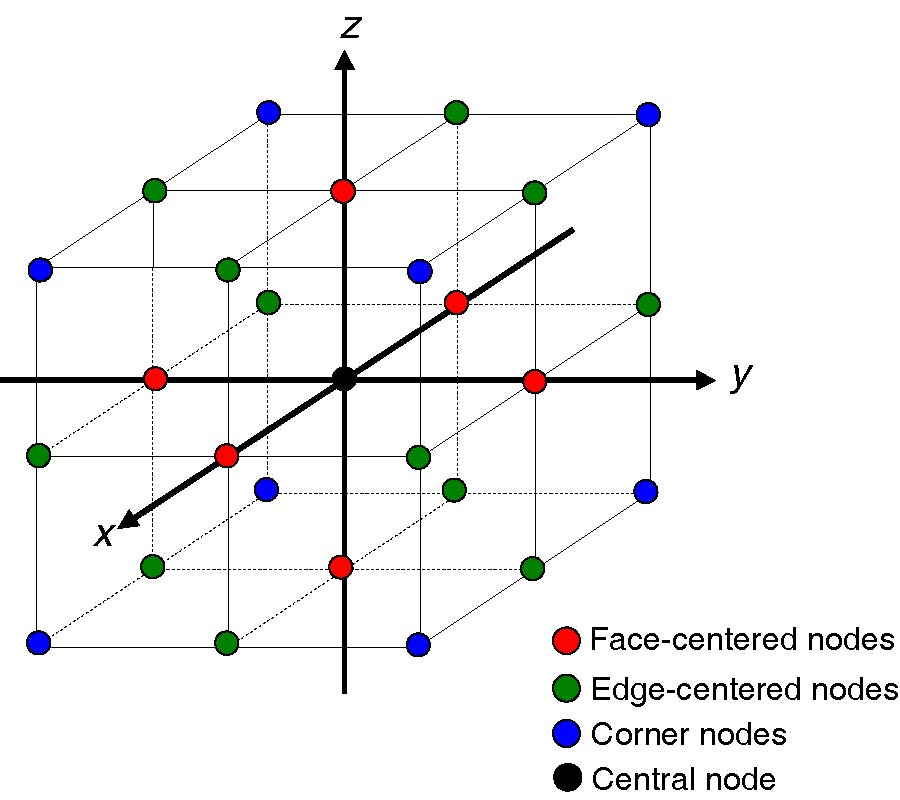# Vol. 142

Front:[PDF file] Back:[PDF file]
Latest Volume
All Volumes
All Issues
2013-08-25

#### Semi-Analytical Solutions of the 3-D Homogeneous Helmholtz Equation by the Method of Connected Local Fields

By Hung-Wen Chang and Sin-Yuan Mu
Progress In Electromagnetics Research, Vol. 142, 159-188, 2013
doi:10.2528/PIER13060906

## Abstract

We advance the theory of the two-dimensional method of connected local fields (CLF) to the three-dimensional cases. CLF is suitable for obtaining semi-analytical solutions of Helmholtz equation. The fundamental building block (cell) of the 3-D CLF is a cube consisting of a central point and twenty six points on the cube's surface. These surface points form three symmetry groups: six on the planar faces, twelve on the edges, and eight on the vertices (corners). The local field within the unit cell is expanded in a truncated spherical Fourier-Bessel series. From this representation we develop a closed-form, 3-D local field expansion (LFE) coefficients that relate the central point to its immediate neighbors. We also compute the CLF-based FD-FD numerical solutions of the 3D Green's function in free space. Compared with the analytic solution, we found that even at a low three points per wavelength spatial sampling, the accumulated phase errors of the CLF 3D Green's function after propagating a distance of ten wavelengths are well under ten percent.

## Citation

Hung-Wen Chang and Sin-Yuan Mu, "Semi-Analytical Solutions of the 3-D Homogeneous Helmholtz Equation by the Method of Connected Local Fields," Progress In Electromagnetics Research, Vol. 142, 159-188, 2013.
doi:10.2528/PIER13060906
http://jpier.org/PIER/pier.php?paper=13060906

## References

1. Chang, H.-W., Y.-H. Wu, and W.-C. Cheng, "Hybrid FD-FD analysis of crossing waveguides by exploiting both the plus and the cross structural symmetry," Progress In Electromagnetics Research, Vol. 103, 217-240, 2010.
doi:10.2528/PIER10030202

2. Yu, C.-P. and H. C. Chang, "Compact finite-difference frequency-domain method for the analysis of two-dimensional photonic crystals," Optics Express, Vol. 12, 1397-1408, 2004.
doi:10.1364/OPEX.12.001397

3. Smith, G. D., Numerical Solution of Partial Differential Equations, 2nd edition, Oxford University Press, 1978.

4. Jo, C.-H., C. Shin, and J. H. Suh, "An optimal 9-point, finite-difference, frequency-space, 2-D scalar wave extrapolator," Geophysics, Vol. 61, 529-537, 1996.
doi:10.1190/1.1443979

5. Smith, v, Numerical Solution of Partial Differential Equations, 2nd edition, Oxford University Press, 1978.

6. Hadley, G. R., "High-accuracy finite-difference equations for dielectric waveguide analysis I: Uniform regions and dielectric interfaces," Journal of Lightwave Technology, Vol. 20, No. 7, 1219-1231, 2002.
doi:10.1109/JLT.2002.800371

7. Hadley, G. R., "High-accuracy finite-difference equations for dielectric waveguide analysis II: Dielectric corners," Journal of Lightwave Technology, Vol. 20, No. 7, 1210-1218, 2002.
doi:10.1109/JLT.2002.800361

8. Tsukerman, I., "Electromagnetic applications of a new finite-difference calculus," IEEE Transactions on Magnetics, Vol. 41, No. 7, 2206-2225, 2005.
doi:10.1109/TMAG.2005.847637

9. Singer, I. and E. Turkel, "Sixth order accurate finite difference schemes for the Helmholtz equation," Journal of Computational Acoustics, Vol. 14, 339-351, 2006.
doi:10.1142/S0218396X06003050

10. Chang, H.-W. and S.-Y. Mu, "Semi-analytical solutions of the 2-D homogeneous Helmholtz equation by the method of connected local fields," Progress In Electromagnetics Research, Vol. 109, 399-424, 2010.
doi:10.2528/PIER10092807

11. Mu, S.-Y. and H.-W. Chang, "Theoretical foundation for the method of connected local fields," Progress In Electromagnetics Research, Vol. 114, 67-88, 2011.

12. Young, D. M. and J. H. Dauwalder, Discrete Representations of Partial Differential Equations, Errors in Digital Computation, Academic Press, New York, 1965.

13. Sutmann, G., "Compact finite difference schemes of sixth order for the Helmholtz equation," Journal of Computational and Applied Mathematics, Vol. 203, 15-31, 2007.
doi:10.1016/j.cam.2006.03.008

14. Fernandes, D. T. and A. F. D. Loula, "Quasi optimal finite difference method for Helmholtz problem on unstructured grids," Int. J. Numer. Meth. Engng., Vol. 82, 1244-1281, 2010.

15. Chang, H.-W. and S.-Y. Mu, "3-D LFE-27 formulae for the method of connected local fields,", OPTIC-2012, OB-SA-BL1, Taipei, Taiwan, 2012.

16. Hall, C. A. and T. A. Porsching, Numerical Analysis of Partial Differential Equations, 248-250, Prentice-Hall, Englewood Cliffs, New Jersey, 1990.

17. Harari, I. and E. Turkel, "Accurate finite difference methods for time-harmonic wave propagation," Journal of Computational Physics, Vol. 119, No. 2, 252-270, 1995.
doi:10.1006/jcph.1995.1134

18. Spotz, W. F. and G. F. Carey, "A high-order compact formulation for the 3D Poisson equation," Numerical Methods for Partial Differential Equations, Vol. 12, No. 2, 235-243, 1996.
doi:10.1002/(SICI)1098-2426(199603)12:2<235::AID-NUM6>3.0.CO;2-R

19. Nehrbass, J. W., J. O. Jevtic, and R. Lee, "Reducing the phase error for finite-difference methods without increasing the order," IEEE Transactions on Antennas and Propagation, Vol. 46, 1194-1201, 1998.
doi:10.1109/8.718575

20. Ernst, O. G. and M. J. Gander, "Why it is difficult to solve Helmholtz problems with classical iterative methods," Lecture Notes in Computational Science and Engineering, Vol. 83, 325-363, 2012.
doi:10.1007/978-3-642-22061-6_10